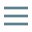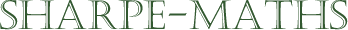# Glossary of terms

## D

•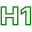### Difference

•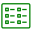The result of a subtraction problem
•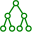The difference between 3 and 9 is 6 or $9 - 3 = 6$
•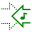•### Directed numbers

•Directed number is a term used to describe integers (mostly). In other words, directed numbers are numbers where some direction is implied. This becomes important in navigation: the journey outward is the opposite of the journey back again, even though it covers the same ground, we can consider one as positive and the other negative.

•It is very important to work carefully when using operations on directed numbers. It is my recommendation when working with directed numbers, that you use implied multiplication with brackets rather than using the $\times$ sign. This is to avoid statements such as $4 \times -3 = -12$ where you have a $\times$ and a $+$ next to each other, as this can lead to confusion. Here is the bracketed alternative: $4\big(-3 \big) = -12$, which means the same thing though, to my mind, it is clearer.

••### Dividend

•This is the number being divided up. In the expression $20 \div 5$, $20$ is the dividend.

•In English, a dividend is the surplus profit of a company, which is divided up after a year of profitable exploitation of its workforce.

In mathematics, it means roughly the same thing, minus the exploitation. A dividend is the number being divided up.

In the identity   $10 \div 2 = 5$,   the dividend is 10.

••### Division

•Division is the operation where we split up a group of things into smaller groups of the same size.
•$18 \div 3 = 6$ is a division problem, involving a dividend ($18$) divided ($\div$) by a divisor ($3$) giving a quotient ($6$).

••### Divisor

•This is the number being divided by. In the expression $20 \div 5$, $5$ is the divisor.

•In the identity, $20 \div 5 = 4$, $5$ is the divisor, $20$ is the dividend and $4$ is the quotient.

•